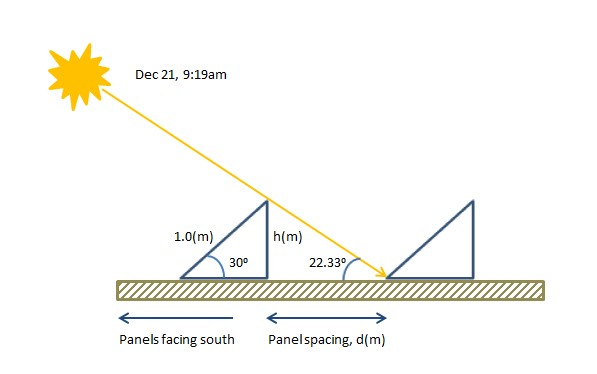# Calculation of Solar Panel Spacing for India (New Dehli)

I have been asked many times that what is the area required for a solar array with a particular rating (such as 20 KW) and I have given a simple calculation method in one of my earlier post. But real life is never so simple. There are a bunch of inputs that we need to get an exact answer. In particular we want to know what is the required spacing between solar panels so that they are not in shade for a particular part of the day (typically 5 or 6 hours) and for this we need a number of inputs such as latitude, longitude, solar noon on the worst day of the year etc. Here we show the sequence of calculations for a typical city in Northern India. Similar calculations would hold true for many cities in Pakistan as well.

Let us first list down the data we have for New Dehli a typical city in Northern India (this data is available on ESRL website).

Latitude: 28.6139
Longitude: 77.2090
Time Zone: +5.5
Worst Solar Day: Dec 21
Start of Solar Charging: 09:19 am
Solar Noon: 12:19 pm
End of Solar Charging: 3:19 pm
Solar Azimuth: 135.5 degrees (Azimuth at 9:19 am, the time solar panel just comes out of the shade)
Solar Inclination: 22.33 degrees (Inclination at 9:19 am, the time solar panel just comes out of the shade)Now lets assume that the length of the solar panel is 1.0 m and it is fixed at an angle of 30 degrees from the horizontal. Then the height can be calculated as:

h = 1.0 * sin(30) = 0.50

Now we are ready to calculate the distance between the panels.

D = h / tan(Inclination) = 0.50 / tan(22.33) = 1.22 m (this is the distance without Solar Azimuth Correction)

d = D * cos(180-Azimuth) = 1.22 * cos(44.5) = 0.87 (this is the distance with Solar Azimuth Correction)

So for a 1.0 m length panel we need to have a separation of about 0.87 m between the panels along the north-south line (panels facing south in the Northern hemisphere). To be on the safe side we can keep the distance a bit higher than this. We can estimate that the total area would be increased by a factor 2.0 (0.87 m is the separation of the panels and 0.87 m is the base of the triangle formed by  the inclined solar panel) or we can simply multiply the area calculated by the simple calculations (for a panel lying flat on the ground) given in the earlier post by a factor of 1.74.

Note: These calculations are valid for solar panels placed on the ground, in straight lines, along the east-west line (facing south). For panels placed on inclined rooftops the calculations would be different (area required would be generally lower). Also note that solar panels are highly sensitive to shading and even if only 10% of the panel is in shade its performance without deteriorate appreciably. Lastly some installations have different panel tilts for winter and summer (latitude +/- 15 degrees) and this needs to be catered in the calculations.#### Author: John (YA)

John has over 20 years of Research and Development experience in the field of Wireless Communications. He has worked for a number of companies around the world including Qualcomm Inc. USA.

4.00 avg. rating (82% score) - 1 vote

## 5 thoughts on “Calculation of Solar Panel Spacing for India (New Dehli)”

1.NISHTHAR says:

Is there any horizontal spacing to be kept between solar modules while installing in tilted roof

1.John (YA) says:

No you do not need spacing on a tilted roof. But the output you get will depend upon the angle and direction of tilt. A south facing, 30 degree tilted roof, would be best for you.

2.Shivraj Deshmukh says:

Estimate 12 kilowatt solar panels area and cast

1.John (YA) says:

Total Wattage = 1kWatt/m2 x Efficiency x Area

12kWatt = 1kWatt/m2 x 0.20 x Area

Area = 60m2

But if the panels are at an inclination on flat ground you may need about twice that area (120m2).

3.Muhammad Ahmed says:

Very good